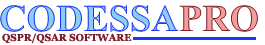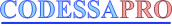Constitutional Topological Geometrical Electrostatic CPSA Quantum-chemical MO-related Thermodynamic

### Balaban’s J index

#### Definition:q - number of edges in the molecular graph

m = (q – n + 1) - the cyclomatic number of the molecular graph

n – number of atoms in the molecular graph

Si - distance sums calculated as the sums over the rows or columns of the topological distance matrix of the molecule, D.

#### References:

1. A. T. Balaban, Chem. Phys. Lett., 1981, 89, 399.
2. A. T. Balaban, Pure and Appl. Chem., 1983, 55, 199.University of Florida 2001. All rights reserved